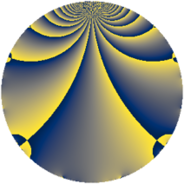# Properties

 Label 429.2.fLevel $429$ Weight $2$ Character orbit 429.f Rep. character $\chi_{429}(131,\cdot)$ Character field $\Q$ Dimension $48$ Newform subspaces $1$ Sturm bound $112$ Trace bound $0$

# Related objects

## Defining parameters

 Level: $$N$$ $$=$$ $$429 = 3 \cdot 11 \cdot 13$$ Weight: $$k$$ $$=$$ $$2$$ Character orbit: $$[\chi]$$ $$=$$ 429.f (of order $$2$$ and degree $$1$$) Character conductor: $$\operatorname{cond}(\chi)$$ $$=$$ $$33$$ Character field: $$\Q$$ Newform subspaces: $$1$$ Sturm bound: $$112$$ Trace bound: $$0$$

## Dimensions

The following table gives the dimensions of various subspaces of $$M_{2}(429, [\chi])$$.

Total New Old
Modular forms 60 48 12
Cusp forms 52 48 4
Eisenstein series 8 0 8

## Trace form

 $$48q - 6q^{3} + 48q^{4} + 14q^{9} + O(q^{10})$$ $$48q - 6q^{3} + 48q^{4} + 14q^{9} - 20q^{12} - 6q^{15} + 8q^{16} - 4q^{22} - 28q^{25} - 12q^{31} + 2q^{33} - 16q^{34} + 26q^{36} + 20q^{37} - 2q^{42} - 50q^{45} - 82q^{48} - 56q^{49} - 20q^{55} - 80q^{58} + 104q^{60} + 16q^{64} - 50q^{66} + 60q^{67} + 6q^{69} + 80q^{70} + 12q^{75} + 10q^{78} + 6q^{81} - 8q^{82} - 52q^{88} - 8q^{91} + 42q^{93} - 92q^{97} + 18q^{99} + O(q^{100})$$

## Decomposition of $$S_{2}^{\mathrm{new}}(429, [\chi])$$ into newform subspaces

Label Dim. $$A$$ Field CM Traces $q$-expansion
$$a_2$$ $$a_3$$ $$a_5$$ $$a_7$$
429.2.f.a $$48$$ $$3.426$$ None $$0$$ $$-6$$ $$0$$ $$0$$

## Decomposition of $$S_{2}^{\mathrm{old}}(429, [\chi])$$ into lower level spaces

$$S_{2}^{\mathrm{old}}(429, [\chi]) \cong$$ $$S_{2}^{\mathrm{new}}(33, [\chi])$$$$^{\oplus 2}$$